# (t), For this question, this system has two inputs. The first input is the velocity of The following figure depicts...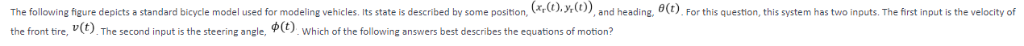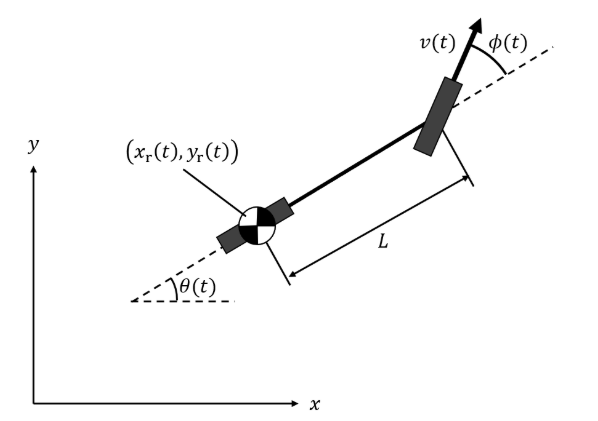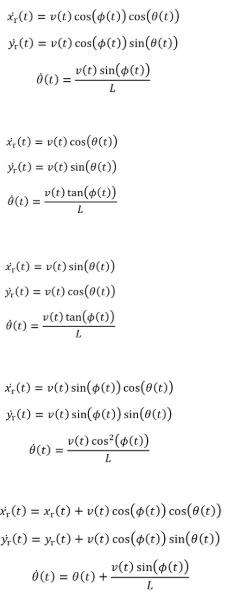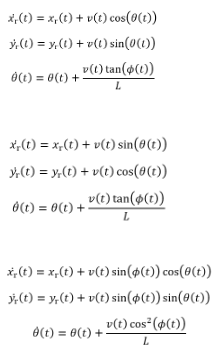(t), For this question, this system has two inputs. The first input is the velocity of The following figure depicts a standard bicycle model used for modeling vehicles. Its state is described by some position, r ф(t) the front tire, v(t). The second input is the steer which of the following answers best describes the equations of motion? the
(xr(t), yr(t))
4(1)-r(t) cos(φ(t)) cos(0(t)) (t)(t) cos(p())sin((t)) 0(1)-v(t)sin(φ(t)) ) (t)cos(o(t) A(t)-v(t) sin(θ (t)) 00-v(t) tan(φ(t)) A(t) 4(t)-v(t) sin(θ(t)) ))cos(o(o) v(t) tan(p(t)) ,(t)-v(t) sin(φ(t)) cos(θ(t)) It(t)-v(t)sin(d(t)) sin(θ(t)) θ(t)s u(t) cos*(φ(t)) +vt) cos(p(t)) cos(()) y(t)-y(t) + u(t) cos(φ(t)) sin(θ (t)) 0(t)s 0(t) + v(t)sin(φ(t))
))+()cos(oo)) ) )+ v)sin(o)) 0(t) + v(t)tan(φ(t)) 6(1) 4(t)-xr(t) + v(t) sin(θ(t)) )-)+v)cos((o) 0Otano )x+v()sin(p()) cos(e()) A(t)-y(t) + v(t) sin(d(t)) sin(θ(t)) 6(t) + v(t)cos2(φ(t)) θ(t)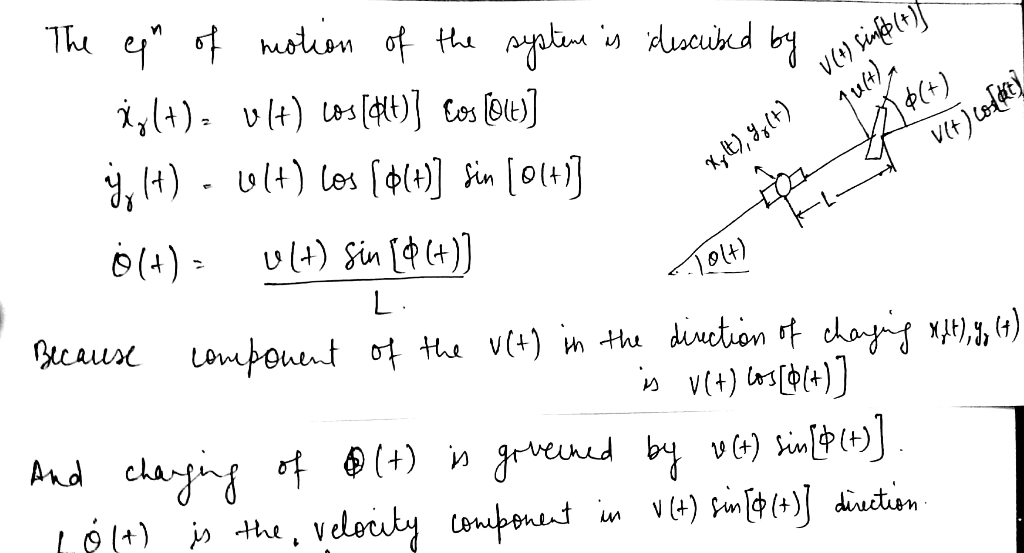##### Add Answer of: (t), For this question, this system has two inputs. The first input is the velocity of The following figure depicts...
More Homework Help Questions Additional questions in this topic.